# MCAT Physical : Motion in Two Dimensions

## Example Questions

← Previous 1 3

### Example Question #91 : Newtonian Mechanics And Motion

A 2kg lead ball is loaded into a spring cannon and the cannon is set at a 45º angle on a platform. The spring has a spring constant of 100N/m and the ball and spring system is compressed by 1m before launch. While the ball is in flight air resistance can be neglected, and the ball finishes its flight by landing at a cushion placed some distance away from the cannon.

What is the horizontal acceleration of the ball during its flight?

-4.9m/s2

-9.8m/s2

0m/s2

9.8m/s2

0m/s2

Explanation:

Without an additional force acting in the horizontal direction during flight (we are told we can neglect air resistance), there is no acceleration. Remember that Newton's second law, F = ma, requires that a force act on an object to produce acceleration. Here, we have no additional force and thus no acceleration.

### Example Question #2 : Motion In Two Dimensions

A ball of mass 3kg is thrown into the air at an angle of 45o above the horizontal, with initial velocity of 15m/s. Instantaneously at the highest point in its motion, the ball comes to rest. Approximately what is the magnitude of acceleration at this point? Assume that air resistance is negligible.

5m/s2

10m/s2

0m/s2

15m/s2

30m/s2

10m/s2

Explanation:

After being thrown, the ball is only acted on by the force of gravity. Since this force is constant throughout the motion, acceleration must also remain constant, and be equal to the gravitational acceleration of 9.8 m/s2 (approximately 10 m/s2)

### Example Question #3 : Motion In Two Dimensions

A daredevil is shot out of a cannon at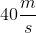at an angle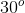above the ground. What will be the maximum height she achieves?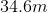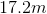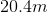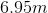Explanation:

We need to calculate the vertical component on the daredevil's velocity using the equation: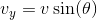Given the angle of trajectory and the initial velocity, we can solve.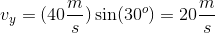At the maximum height, the daredevil will have zero vertical velocity. Using this as the final vertical velocity, we can calculate the distance traveled using the appropriate kinematics equation.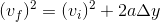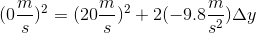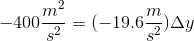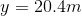### Example Question #4 : Motion In Two Dimensions

Two children are playing with sleds on a snow-covered hill. Sam weighs 50kg, and his sled weighs 10kg. Sally weighs 40kg, and her sled weighs 12kg. When they arrive, they climb up the hill using boots. Halfway up the 50-meter hill, Sally slips and rolls back down to the bottom. Sam continues climbing, and eventually Sally joins him at the top.

They then decide to sled down the hill, but disagree about who will go first.

Scenario 1:

Sam goes down the hill first, claiming that he will reach a higher velocity. If Sally had gone first, Sam says they could collide.

Scenario 2:

Sally goes down the hill first, claiming that she will experience lower friction and thus reach a higher velocity. If Sam had gone first, Sally says they could collide.

Scenario 3:

Unable to agree, Sam and Sally tether themselves with a rope and go down together.

In Scenario 3, before they begin moving, Sam is hanging down the hill facing forward, and Sally has fixed her shoes in the snow to keep her and Sam from beginning down the hill together. If the hill is at a 30o angle to the horizontal, and Sam is stationary but tied to Sally by a rope, how much force does Sam experience down the surface of the hill?

450J

300kJ

300J

150J

600J

300J

Explanation:

Sam will experience force down the surface of the inclined plane as per the equation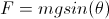.

F = (60kg)(10m/s2) * sin(30o) = 300J

### Example Question #1 : Motion In Two Dimensions

Two children are playing with sleds on a snow-covered hill. Sam weighs 50kg, and his sled weighs 10kg. Sally weighs 40kg, and her sled weighs 12kg. When they arrive, they climb up the hill using boots. Halfway up the 50-meter hill, Sally slips and rolls back down to the bottom. Sam continues climbing, and eventually Sally joins him at the top.

They then decide to sled down the hill, but disagree about who will go first.

Scenario 1:

Sam goes down the hill first, claiming that he will reach a higher velocity. If Sally had gone first, Sam says they could collide.

Scenario 2:

Sally goes down the hill first, claiming that she will experience lower friction and thus reach a higher velocity. If Sam had gone first, Sally says they could collide.

Scenario 3:

Unable to agree, Sam and Sally tether themselves with a rope and go down together.

In Scenario 3, before they begin moving, Sam is hanging down the hill face first, and Sally has fixed her shoes in the snow to keep her and Sam from beginning down the hill together. If the hill is at a 30o angle to the horizontal, and Sam is stationary but tied to Sally by a rope, how much tension is in the rope?

600J

450J

150J

300J

900J

300J

Explanation:

Sam is pulling down the inclinced plane with a force given by the equation.

F = 60kg * 10m/s2 * sin(30) = 300J

Tension in the rope will be equal to this value, as these forces must cancel to result in a net force of zero.

### Example Question #6 : Motion In Two Dimensions

Two children are playing with sleds on a snow-covered hill. Sam weighs 50kg, and his sled weighs 10kg. Sally weighs 40kg, and her sled weighs 12kg. When they arrive, they climb up the hill using boots. Halfway up the 50-meter hill, Sally slips and rolls back down to the bottom. Sam continues climbing, and eventually Sally joins him at the top.

They then decide to sled down the hill, but disagree about who will go first.

Scenario 1:

Sam goes down the hill first, claiming that he will reach a higher velocity. If Sally had gone first, Sam says they could collide.

Scenario 2:

Sally goes down the hill first, claiming that she will experience lower friction and thus reach a higher velocity. If Sam had gone first, Sally says they could collide.

Scenario 3:

Unable to agree, Sam and Sally tether themselves with a rope and go down together.

Which of the following expressions shows the force that Sally and Sam would feel down the hill at a 30o incline if they both rode on Sam’s sled together?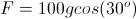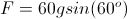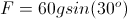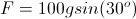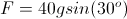Explanation:

This is a tricky question. The total mass of Sally, Sam, and Sam's sled is 100kg. The force down the incline is.

### Example Question #7 : Motion In Two Dimensions

A 2kg box is at the top of a frictionless ramp at an angle of 60o. The top of the ramp is 30m above the ground. The box is sitting still while at the top of the ramp, and is then released.

Which of these statements is true?

The force on the box is greater at the bottom of the ramp than at the top of the ramp

The normal force is always perpendicular to the angle of the ramp

As the angle of the ramp is decreases, the acceleration on the box due to gravity decreases

The box has a greater acceleration at the bottom of the ramp

The normal force is always perpendicular to the angle of the ramp

Explanation:

Normal force will always be perpendicular to the surface in question.

The acceleration due to gravity does not change with ramp angle. It is the force due to gravity that changes with ramp angle. The acceleration on an object due to gravity is a constant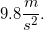### Example Question #1 : Understanding Normal Force

A 2kg box is at the top of a frictionless ramp at an angle of 60o. The top of the ramp is 30m above the ground. The box is sitting still while at the top of the ramp, and is then released.

If the angle of the ramp is decreased, which of the following statements is false?

The normal force will decrease

The time to reach the ground will increase

The force due to gravity will decrease

The net force on the box will decrease

The normal force will decrease

Explanation:

When given a question about the angle of a ramp, compare it to the extreme angles: 0o and 90o.

1. When the ramp has an angle of 0o, the net force 0. The force due to gravity must equal the normal force; thus the normal force is at a maximum value.

2. When the angle of the ramp is 90o, the full force of gravity is experienced by the box, and there is no normal force. The net force is equal to the force of gravity. Remember that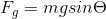, so then theta is 90o, force of gravity is at a maximum.

When we decrease the angle of the ramp, we get closer to scenario 1. As a result, we can conclude that the normal force on the box increases, rather than decreases.

### Example Question #8 : Motion In Two Dimensions

A 2kg lead ball is loaded into a spring cannon and the cannon is set at a 45º angle on a platform. The spring has a spring constant of 100N/m and the ball and spring system is compressed by 1m before launch. While the ball is in flight air resistance can be neglected, and the ball finishes its flight by landing at a cushion placed some distance away from the cannon.

Find the horizontal component of velocity once the ball has left the cannon.

7.1m/s

9.2m/s

8.5m/s

4.9m/s

7.1m/s

Explanation:

This asks us to understand the vector components of velocity. Remember that the final velocity is the hypotenuse of a triangle (solved to be 10m/s in the previous problem), and that by knowing the hypotenuse value we can solve for the horizontal component by using cosine.

vx = (10m/s)(cos(45o)) = 7.1m/s

### Example Question #9 : Motion In Two Dimensions

A 2kg lead ball is loaded into a spring cannon and the cannon is set at a 45º angle on a platform. The spring has a spring constant of 100N/m and the ball and spring system is compressed by 1m before launch. While the ball is in flight air resistance can be neglected, and the ball finishes its flight by landing at a cushion placed some distance away from the cannon.

What is the initial vertical component of velocity of the ball?

7.1m/s

2.3m/s

7.9m/s

5.2m/s